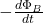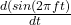Question

Scientific work is currently under way to determine whether weak oscillating magnetic fields can affect human health. For example, one study found that driv- ers of trains had a higher incidence of blood cancer than other railway workers, possibly due to long expo- sure to mechanical devices in the train engine cab. Consider a magnetic field of magnitude 1.00 3 1023 T, oscillating sinusoidally at 60.0 Hz. If the diameter of a red blood cell is 8.00 mm, determine the maximum emf that can be generated around the perimeter of a cell in this field.

1.Thunguyet

fem = 7.58 10⁻⁵ V

Explanation:

For this exercise we use Faraday’s law

fem =the magnetic flux is

Ф_B = B. A = B A cos θ

Tje bold are vectros.  Suppose the case where the normal to the surface of the red blood cell is parallel to the field, therefore the angle is zero and the cos 0 = 1

The red blood cell area is

A =π r²

indicate that the diameter is r = 8.00 mm = 8.00 10⁻³ m

the magnetic field has a frequency of f=60 Hz, and B₀ = 1.00 10⁻³T,  therefore we can write it

B = B₀ sin (wt) = B₀ sin( 2π f t)

we substitute

fem = – A dB / dt

fem = – A B₀fem = – π r² Bo (2πf  cos 2πft)

the maximum electromotive force occurs when the function is ±1

fem = 2 π² r² B₀ f

let’s calculate

fem = 2π²  (8.00 10⁻³)²  1.00 10⁻³ 60

fem = 7.58 10⁻⁵ V